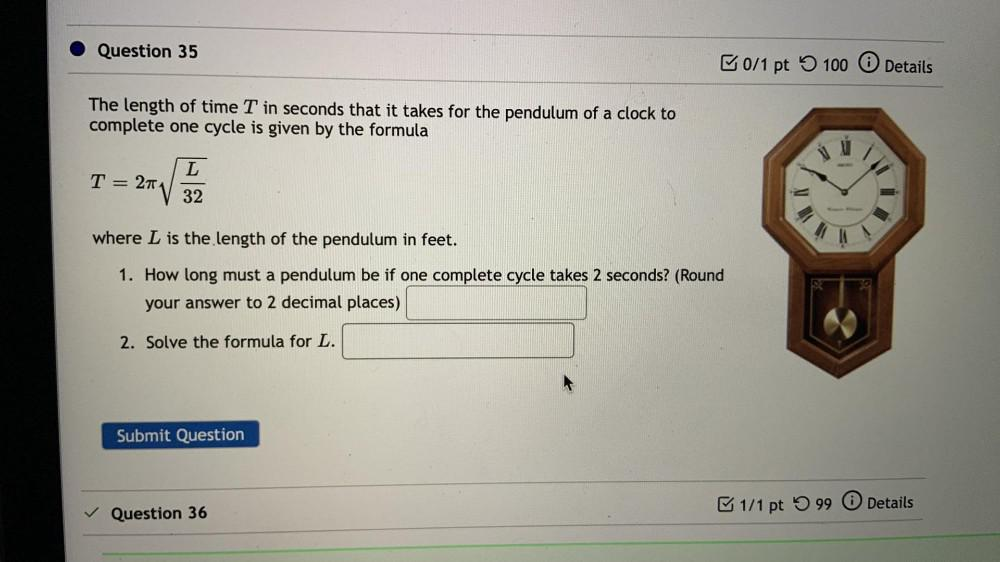Question:

# Question 35 0/1 pt 100 0 Details The length of time T in seconds that it takes for the pendulum of a clock to complete one cycleQuestion 35 0/1 pt 100 0 Details The length of time T in seconds that it takes for the pendulum of a clock to complete one cycle is given by the formula L T 27 32 where L is the length of the pendulum in feet. 1. How long must a pendulum be if one complete cycle takes 2 seconds? (Round your answer to 2 decimal places) 2. Solve the formula for L. Submit Question 1/1 pt 99 0 Details Question 36